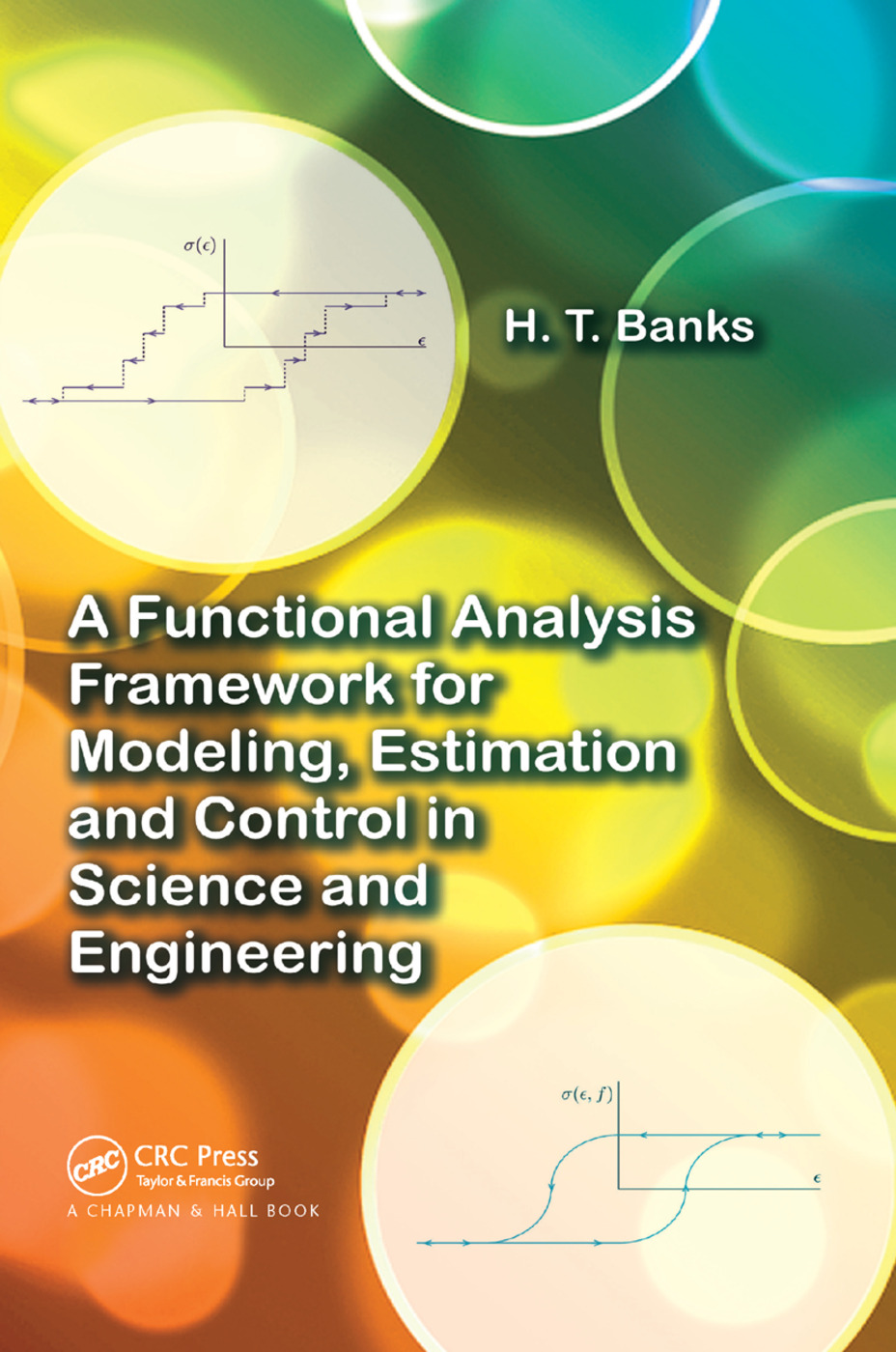A Functional Analysis Framework for Modeling, Estimation and Control in Science and Engineering

1st Edition

Chapman and Hall/CRC

280 pages | 9 B/W Illus.

New in Paperback: 9781138374638
pub: 2018-09-18
\$71.00
x
Hardback: 9781439880838
pub: 2012-06-18
\$95.95
x
eBook (VitalSource) : 9780429086380
pub: 2012-06-18
from \$35.50

FREE Standard Shipping!

Description

A Modern Framework Based on Time-Tested Material

A Functional Analysis Framework for Modeling, Estimation and Control in Science and Engineering presents functional analysis as a tool for understanding and treating distributed parameter systems. Drawing on his extensive research and teaching from the past 20 years, the author explains how functional analysis can be the basis of modern partial differential equation (PDE) and delay differential equation (DDE) techniques.

Recent Examples of Functional Analysis in Biology, Electromagnetics, Materials, and Mechanics

Through numerous application examples, the book illustrates the role that functional analysis—a classical subject—continues to play in the rigorous formulation of modern applied areas. The text covers common examples, such as thermal diffusion, transport in tissue, and beam vibration, as well as less traditional ones, including HIV models, uncertainty in noncooperative games, structured population models, electromagnetics in materials, delay systems, and PDEs in control and inverse problems. For some applications, computational aspects are discussed since many problems necessitate a numerical approach.

Reviews

"The book under review has the valuable advantage of being of interest to both mathematicians and engineers. … appropriate tools are carefully introduced and discussed in detail, and they are readily applied to practical situations related to the models derived from the generic examples. The main thrust of the book consists of those parts and topics of functional analysis that are fundamental to rigorous discussions of practical differential equations and delay systems as they arise in diverse applications and in particular in control and estimation."

—Larbi Berrahmoune, Mathematical Reviews, May 2013

Introduction to Functional Analysis in Applications

Example 1: Heat Equation

Some Preliminaries: Hilbert, Banach, and Other Spaces Useful in Operator Theory

Example 2: General Transport Equation

Example 3: Delay Systems–Insect/Insecticide Models

Example 4: Probability Measure Dependent Systems — Maxwell’s Equations

Example 5: Structured Population Models

Semigroups and Infinitesimal Generators

Basic Principles of Semigroups

Infinitesimal Generators

Generators

Introduction to Generation Theorems

Hille-Yosida Theorems

Results from the Hille-Yosida Proof

Corollaries to Hille-Yosida

Lumer-Phillips and Dissipative Operators

Examples Using Lumer-Phillips Theorem

Dual Spaces and Strong, Weak, and Weak* Topologies

Examples of Spaces and Their Duals

Gelfand Triple, Sesquilinear Forms, and Lax-Milgram

Example 6: The Cantilever Beam

The Beam Equation in the Form x derivative = Ax + F

Gelfand Triples

Sesquilinear Forms

Lax-Milgram (Bounded Form)

Lax-Milgram (Unbounded Form)

Summary Remarks and Motivation

Analytic Semigroups

Example 1: The Heat Equation (again)

Example 2: The Transport Equation (again)

Example 6: The Beam Equation (again)

Summary of Results on Analytic Semigroup Generation by Sesquilinear Forms

Tanabe Estimates (on "Regular Dissipative Operators")

Infinitesimal Generators in a General Banach Space

Abstract Cauchy Problems

General Second-Order Systems

Introduction to Second-Order Systems

Results for σ2 V-elliptic

Results for σ2 H-semielliptic

Stronger Assumptions for σ2

Weak Formulations for Second-Order Systems

Model Formulation

Discussion of the Model

Theorems 9.1 and 9.2: Proofs

Inverse or Parameter Estimation Problems

Approximation and Convergence

Some Further Remarks

"Weak" or "Variational Form"

Finite Element Approximations and the Trotter-Kato Theorems

Finite Elements

Trotter-Kato Approximation Theorem

Delay Systems: Linear and Nonlinear

Linear Delay Systems and Approximation

Modeling of Viral Delays in HIV Infection Dynamics

Nonlinear Delay Systems

State Approximation and Convergence for Nonlinear Delay Systems

Fixed Delays versus Distributed Delays

Weak* Convergence and the Prohorov Metric in Inverse Problems

Populations with Aggregate Data, Uncertainty, and PBM

A Prohorov Metric Framework for Inverse Problems

Metrics on Probability Spaces

Example 5: The Growth Rate Distribution Model and Inverse Problem in Marine Populations

The Prohorov Metric in Optimization and Optimal Design Problems

Two Player Min-Max Games with Uncertainty

Optimal Design Techniques

Generalized Curves and Relaxed Controls of Variational Theory

Preisach Hysteresis in Smart Materials

NPML and Mixing Distributions in Statistical Estimation

Control Theory for Distributed Parameter Systems

Motivation

Abstract Formulation

Infinite Dimensional LQR Control: Full State Feedback

The Finite Horizon Control Problem

The Infinite Horizon Control Problem

Families of Approximate Control Problems

The Finite Horizon Problem Approximate Control Gains

The Infinite Horizon Problem Approximate Control Gains

References

Index